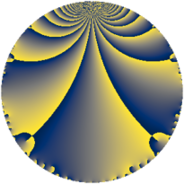# Properties

 Label 8470.2.a.bbLevel $8470$ Weight $2$ Character orbit 8470.a Self dual yes Analytic conductor $67.633$ Analytic rank $1$ Dimension $1$ CM no Inner twists $1$

# Related objects

Show commands: Magma / PariGP / SageMath

## Newspace parameters

comment: Compute space of new eigenforms

[N,k,chi] = [8470,2,Mod(1,8470)]

mf = mfinit([N,k,chi],0)

lf = mfeigenbasis(mf)

from sage.modular.dirichlet import DirichletCharacter

H = DirichletGroup(8470, base_ring=CyclotomicField(2))

chi = DirichletCharacter(H, H._module([0, 0, 0]))

N = Newforms(chi, 2, names="a")

//Please install CHIMP (https://github.com/edgarcosta/CHIMP) if you want to run this code

chi := DirichletCharacter("8470.1");

S:= CuspForms(chi, 2);

N := Newforms(S);

 Level: $$N$$ $$=$$ $$8470 = 2 \cdot 5 \cdot 7 \cdot 11^{2}$$ Weight: $$k$$ $$=$$ $$2$$ Character orbit: $$[\chi]$$ $$=$$ 8470.a (trivial)

## Newform invariants

comment: select newform

sage: f = N # Warning: the index may be different

gp: f = lf \\ Warning: the index may be different

 Self dual: yes Analytic conductor: $$67.6332905120$$ Analytic rank: $$1$$ Dimension: $$1$$ Coefficient field: $$\mathbb{Q}$$ Coefficient ring: $$\mathbb{Z}$$ Coefficient ring index: $$1$$ Twist minimal: yes Fricke sign: $$1$$ Sato-Tate group: $\mathrm{SU}(2)$

## $q$-expansion

comment: q-expansion

sage: f.q_expansion() # note that sage often uses an isomorphic number field

gp: mfcoefs(f, 20)

 $$f(q)$$ $$=$$ $$q + q^{2} + q^{3} + q^{4} - q^{5} + q^{6} - q^{7} + q^{8} - 2 q^{9}+O(q^{10})$$ q + q^2 + q^3 + q^4 - q^5 + q^6 - q^7 + q^8 - 2 * q^9 $$q + q^{2} + q^{3} + q^{4} - q^{5} + q^{6} - q^{7} + q^{8} - 2 q^{9} - q^{10} + q^{12} + q^{13} - q^{14} - q^{15} + q^{16} - 6 q^{17} - 2 q^{18} + 7 q^{19} - q^{20} - q^{21} + 3 q^{23} + q^{24} + q^{25} + q^{26} - 5 q^{27} - q^{28} - 6 q^{29} - q^{30} + 2 q^{31} + q^{32} - 6 q^{34} + q^{35} - 2 q^{36} - 4 q^{37} + 7 q^{38} + q^{39} - q^{40} - q^{42} + 4 q^{43} + 2 q^{45} + 3 q^{46} - 6 q^{47} + q^{48} + q^{49} + q^{50} - 6 q^{51} + q^{52} - 5 q^{54} - q^{56} + 7 q^{57} - 6 q^{58} - 3 q^{59} - q^{60} - 2 q^{61} + 2 q^{62} + 2 q^{63} + q^{64} - q^{65} - 4 q^{67} - 6 q^{68} + 3 q^{69} + q^{70} - 2 q^{72} + 4 q^{73} - 4 q^{74} + q^{75} + 7 q^{76} + q^{78} - 17 q^{79} - q^{80} + q^{81} - 9 q^{83} - q^{84} + 6 q^{85} + 4 q^{86} - 6 q^{87} - 18 q^{89} + 2 q^{90} - q^{91} + 3 q^{92} + 2 q^{93} - 6 q^{94} - 7 q^{95} + q^{96} - 10 q^{97} + q^{98}+O(q^{100})$$ q + q^2 + q^3 + q^4 - q^5 + q^6 - q^7 + q^8 - 2 * q^9 - q^10 + q^12 + q^13 - q^14 - q^15 + q^16 - 6 * q^17 - 2 * q^18 + 7 * q^19 - q^20 - q^21 + 3 * q^23 + q^24 + q^25 + q^26 - 5 * q^27 - q^28 - 6 * q^29 - q^30 + 2 * q^31 + q^32 - 6 * q^34 + q^35 - 2 * q^36 - 4 * q^37 + 7 * q^38 + q^39 - q^40 - q^42 + 4 * q^43 + 2 * q^45 + 3 * q^46 - 6 * q^47 + q^48 + q^49 + q^50 - 6 * q^51 + q^52 - 5 * q^54 - q^56 + 7 * q^57 - 6 * q^58 - 3 * q^59 - q^60 - 2 * q^61 + 2 * q^62 + 2 * q^63 + q^64 - q^65 - 4 * q^67 - 6 * q^68 + 3 * q^69 + q^70 - 2 * q^72 + 4 * q^73 - 4 * q^74 + q^75 + 7 * q^76 + q^78 - 17 * q^79 - q^80 + q^81 - 9 * q^83 - q^84 + 6 * q^85 + 4 * q^86 - 6 * q^87 - 18 * q^89 + 2 * q^90 - q^91 + 3 * q^92 + 2 * q^93 - 6 * q^94 - 7 * q^95 + q^96 - 10 * q^97 + q^98

## Embeddings

For each embedding $$\iota_m$$ of the coefficient field, the values $$\iota_m(a_n)$$ are shown below.

For more information on an embedded modular form you can click on its label.

comment: embeddings in the coefficient field

gp: mfembed(f)

Label $$\iota_m(\nu)$$ $$a_{2}$$ $$a_{3}$$ $$a_{4}$$ $$a_{5}$$ $$a_{6}$$ $$a_{7}$$ $$a_{8}$$ $$a_{9}$$ $$a_{10}$$
1.1
 0
1.00000 1.00000 1.00000 −1.00000 1.00000 −1.00000 1.00000 −2.00000 −1.00000
 $$n$$: e.g. 2-40 or 990-1000 Significant digits: Format: Complex embeddings Normalized embeddings Satake parameters Satake angles

## Atkin-Lehner signs

$$p$$ Sign
$$2$$ $$-1$$
$$5$$ $$1$$
$$7$$ $$1$$
$$11$$ $$-1$$

## Inner twists

This newform does not admit any (nontrivial) inner twists.

## Twists

By twisting character orbit
Char Parity Ord Mult Type Twist Min Dim
1.a even 1 1 trivial 8470.2.a.bb yes 1
11.b odd 2 1 8470.2.a.m 1

By twisted newform orbit
Twist Min Dim Char Parity Ord Mult Type
8470.2.a.m 1 11.b odd 2 1
8470.2.a.bb yes 1 1.a even 1 1 trivial

## Hecke kernels

This newform subspace can be constructed as the intersection of the kernels of the following linear operators acting on $$S_{2}^{\mathrm{new}}(\Gamma_0(8470))$$:

 $$T_{3} - 1$$ T3 - 1 $$T_{13} - 1$$ T13 - 1 $$T_{17} + 6$$ T17 + 6 $$T_{19} - 7$$ T19 - 7

## Hecke characteristic polynomials

$p$ $F_p(T)$
$2$ $$T - 1$$
$3$ $$T - 1$$
$5$ $$T + 1$$
$7$ $$T + 1$$
$11$ $$T$$
$13$ $$T - 1$$
$17$ $$T + 6$$
$19$ $$T - 7$$
$23$ $$T - 3$$
$29$ $$T + 6$$
$31$ $$T - 2$$
$37$ $$T + 4$$
$41$ $$T$$
$43$ $$T - 4$$
$47$ $$T + 6$$
$53$ $$T$$
$59$ $$T + 3$$
$61$ $$T + 2$$
$67$ $$T + 4$$
$71$ $$T$$
$73$ $$T - 4$$
$79$ $$T + 17$$
$83$ $$T + 9$$
$89$ $$T + 18$$
$97$ $$T + 10$$